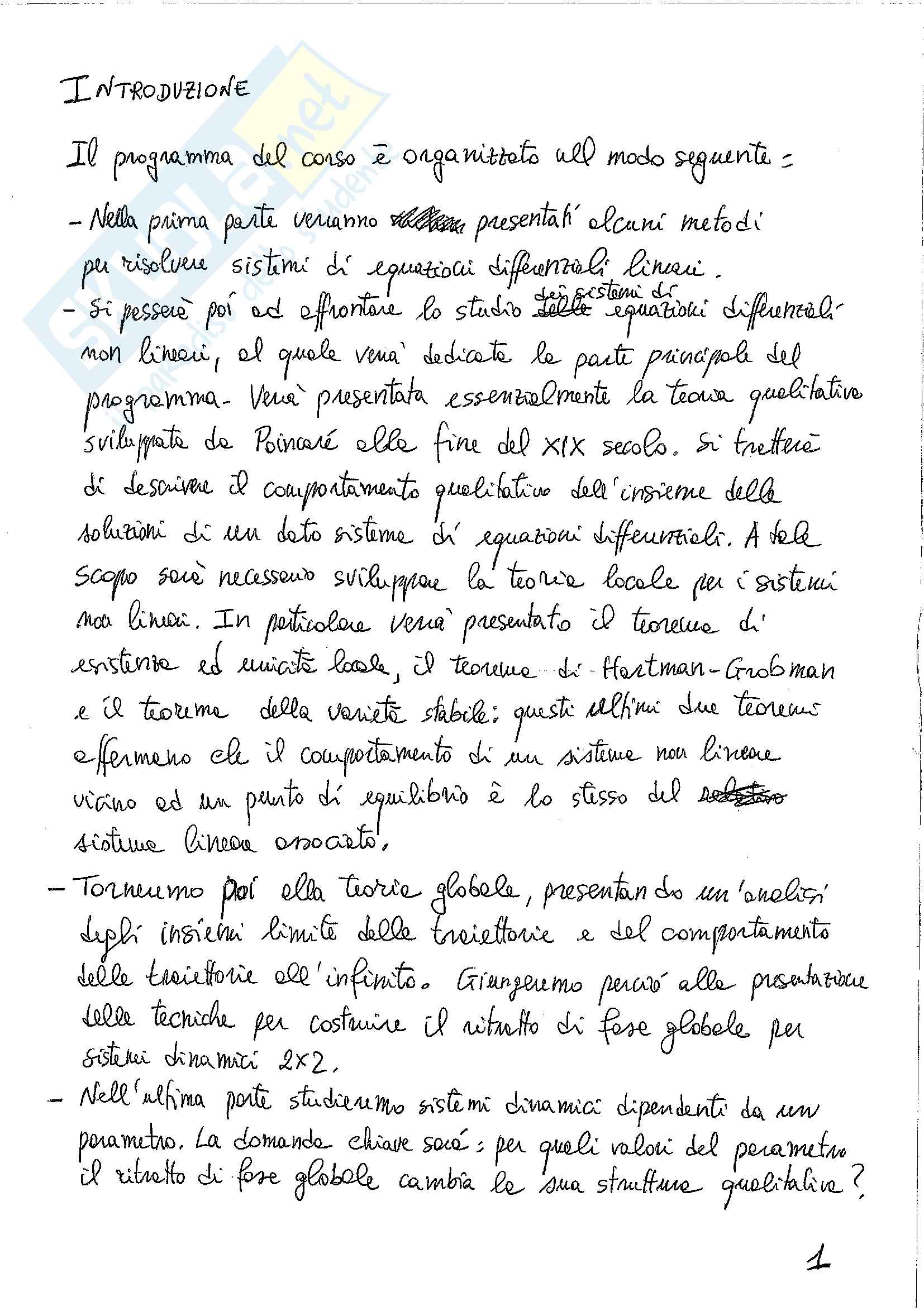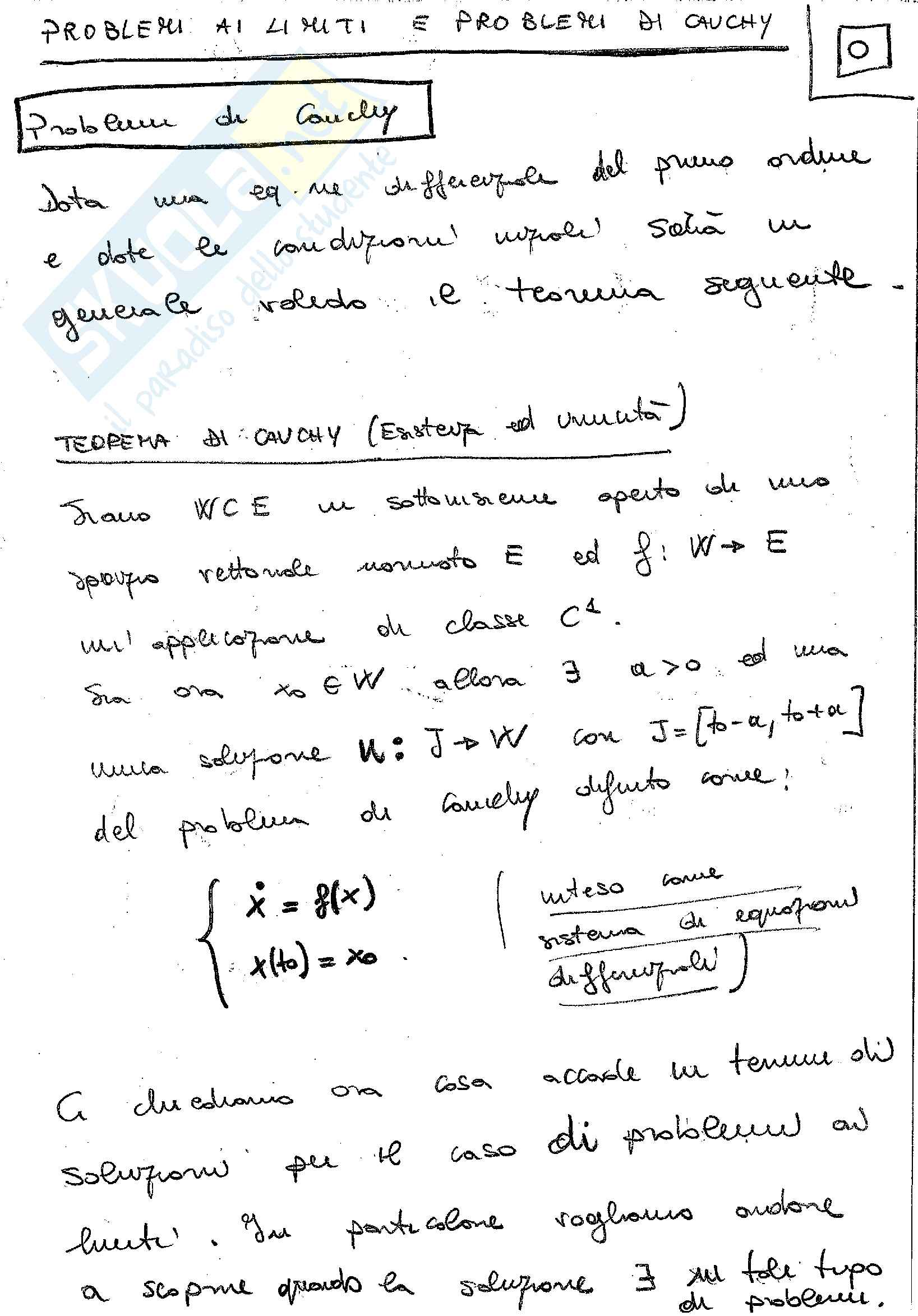Che materia stai cercando?

# Center Manifold Method and Normal Form Theory

The Center Manifold Method
- Existence of an invariant manifold
- Dependence of the CM on parameters
- Reduction process
- Example 1: a static bifurcation
- Example 2: a dynamical bifurcation
The Normal Form Theory
- Scope: to use a smooth nonlinear coordinate transformation, in order to put the bifurcation... Vedi di più

Esame di Sistemi dinamici e teoria della biforcazione docente Prof. A. Luongo

Anteprima

### ESTRATTO DOCUMENTO

Center manifold: coordinates

active and passive

Cartesian equations for the CM:

=

x h x

( , )

µ

s c

Tangency requirements: = = =

h 0 0 0 h 0 0 0 h 0 0 0

( , ) , ( , ) , ( , ) .

x µ

x

The equation expresses the stable variables as (unknown) functions of the

s

x x x

critical variables ; therefore are also said and

active coordinates,

c c s

passive coordinates.

x

Time derivative of :

s x

The passive character of also holds for time-derivatives. By using the

s

chain rule and the upper partition of the equations of motion:

d

= =

x h x h x x

( , ) ( , )

µ µ

x

s c c c

d t

= +

h x J x f x h x

( , )( ( , ( ), ))

µ µ

x c c c c c c 6

Equation for the center manifold:

The lower-partition of the equation of motion supplies the equation for

determining :

c + = +

h x J x f x h x J h x f x h x

( , )( ( , ( ), )) ( ) ( , ( ), )

µ µ µ

x c c c c c c s c s c c

This equation can be solved, e.g., by using power series expansions, (starting

from degree-2 polynomial, due to the required tangency):

2 3

= + + =

h x z z z x

( , ) , : { , }

µ α α µ

2 3

c c

Bifurcation equations:

The upper-partition governs the dynamics on :

c

= +

x J x f x h x

( , ( ), )

µ

c c c c c c 7

Example 1: a static bifurcation

Nonlinear, 2-dimensional system:  

      3

µ

+

0

x x xy cx

 

= +

   

   

− 2

0 1

     

y y  

bx

Critical point, critical and stable coordinates:

µ = = =

x x

0, { }, { }

x y

c c s

Equation for the Center Manifold:

⇒ 3 2

µ µ µ µ µ

= + + = − +

( , ) ( , )[ ( , ) ] ( , )

y h x h x x xh x cx h x bx

x

Power series expansion: 2 2 3

µ α α µ α µ α

= = + + + + +

( , ) { } { }

y h x x x x

20 11 02 30 8

Zeroing separately the different powers:

2 α

− + =

: 0

x b

20

µ α

− =

: 0

x 11

2

µ α

− =

: 0

02

By solving for coefficients, at the lowest order, we have:

α’s 3

2 µ

= + O( ( , ) )

y bx x

Bifurcation equation: 3

µ

= + +

( )

x x b c x

If a supercritical (stable) pitchfork occurs; if a sub-critical

b+c<0, b+c>0,

(unstable) pitchfork occurs; if higher-order terms must be evaluated.

b+c=0, 9

Example 2: a dynamical bifurcation

3-dimensional nonlinear system:  

    

µ

1 0

x x xz

 

    

µ

= +

1 0

y y yz

 

    

      

2 2

0 0 1

z z

     + +

 

x kxy y

Equation for CM: 2 2

α α α α µ α µ

= + + + + + O(3)

z x y xy x y

1 2 3 4 5

(passive coordinate), transformed:

z-equation 2 2

α α α α µ α µ

− + − + −

2( ) ( )

xy x y x y

2 1 3 5 4

2 2 2 2

α α α α µ α µ

= − + + + + + + + +

( ) O(3)

x y xy x y x kxy y

1 2 3 4 5 10

Zeroing separately the different powers in the z-equation:

 2 α α

+ − = 

: 1 0

x α = −

1 / 5

k

3 1

 1

2 α α α

− + − = = +

 : 1 0

y 1 / 5

k

3 2 2

 

α α α α

 

− + − = =

: 2( ) 0 / 5

xy k k

2 1 3 3

 

µ α α α

+ = =

: 0 0

x

 

4 5 4

 

α

µ α α =

− = 0

: 0

y 

 5

5 4

Bifurcation equations:

 2 2

µ

 = − + − + + +

[(1 / 5) / 5 (1 / 5) ]

x x y x k x k xy k y

 2 2

 µ

= − + − + + +

[(1 / 5) / 5 (1 / 5) ]

y y x y k x k xy k y

≠ =

z

0 0

k k

Note: if , the inertia contributes to the motion; if , contributes

z

statically. 11

6.THE NORMAL FORM THEORY

• Scope:

To use a smooth in order to put the

nonlinear coordinate transformation,

bifurcation equation in the simplest form.

• Algorithm: = +

x Jx f x

( )

Equations of motion: = +

y Jy g y

( )

Transformed Equations: = +

x y h y

( )

Near-identity transformation:

x µ.

where (possibly) includes the dummy variables 12

h(y)

Transformed equation in the unknown:

o By differentiating the nearly-identity transformation:

= +

x I h y y

[ ( )]

y

the equation of motion is transformed into:

+ + = + + +

I h y Jy g y J y h y f y h y

[ ( )][ ( )] [ ( )] ( ( ))

y

or: − = + − +

h y Jy Jh y f y h y I h y g y

( ) ( ) ( ( )) [ ( )] ( )

y y

h(y) g(y).

which is a differential equation for for any given A series solution is

often necessary. 13

Series solution:

o  

 

  f y

f y ( )

( )

f y

( ) 3

2  

 

  = + +

g y g y g y

( ) ( ) ( )

 

 

  2 3

     

h y

( ) h y

( ) h y

  ( )

   

2 3

Chain of equations:

o − = −

h y Jy Jh y f y g y

( ) ( ) ( ) ( )

y

2, 2 2 2

− = + − −

h y Jy Jh y f y f y h y h y g y g y

( ) ( ) ( ) ( ) ( ) ( ) ( ) ( )

y y y

3, 3 3 2, 2 2, 2 3

i.e.: ˆ

= − =

h y f y g y

( ) ( ) ( ), 2,3,

k

k k k 14

Homogeneous polynomial of k-degree:

o M M M

k k k

∑ ∑ ∑

ˆ α β γ

= = =

f y p y g y p y h y p y

( ) ( ), ( ) ( ), ( ) ( )

k km km k km km k km km

= = =

1 1 1

m m m

Zeroing the independent monomials:

o = −

L γ α β

k k k k

## J

where (if is diagonal): N N

∑ ∑

λ λ

= Λ Λ = − =

L diag[ ], : ( ),

m m k

k i i j j i j

= =

1 1

j j

Resonance:

o Λ

λ ω ω =

= ± ± 0

0, 0, , , ,

i i L

Since , for some (i.e. is singular);

i k

i

1 2

j ≠ 0

β

hence, must be taken for compatibility, and resonant terms survive in

k

the normal form!!! 15

• Example 1: System independent of parameters

System a Hopf bifurcation:

at

o  

  3 2 2 3

    α α α α

ω + + +

x x x x xx x

0

i x 31 32 33 34

  α

= + ∈

 

, ,

x

 

   

ω

− 3 2 2 3

   

0 i x α α α α

 

x + + +

x x x xx x

 

31 32 33 34

Normal form:

o 3 2 2 3

ω β β β β

= + + + +

y i y y y y yy y

31 32 33 34

Near-identity transformation:

o 3 2 2 3

γ γ γ γ

= + + + +

x y y y y yy y

31 32 33 34 16

PAGINE

25

PESO

160.96 KB

AUTORE

PUBBLICATO

+1 anno fa

### DESCRIZIONE DISPENSA

The Center Manifold Method
- Existence of an invariant manifold
- Dependence of the CM on parameters
- Reduction process
- Example 1: a static bifurcation
- Example 2: a dynamical bifurcation
The Normal Form Theory
- Scope: to use a smooth nonlinear coordinate transformation, in order to put the bifurcation equation in the simplest form.
- Algorithm
- Example 1: System independent of parameters
- Example 2: System dependent on a parameter
- Example 3: Non-diagonalizable system.

DETTAGLI
Corso di laurea: Corso di laurea magistrale in ingegneria civile
SSD:
Università: L'Aquila - Univaq
A.A.: 2011-2012

I contenuti di questa pagina costituiscono rielaborazioni personali del Publisher Atreyu di informazioni apprese con la frequenza delle lezioni di Sistemi dinamici e teoria della biforcazione e studio autonomo di eventuali libri di riferimento in preparazione dell'esame finale o della tesi. Non devono intendersi come materiale ufficiale dell'università L'Aquila - Univaq o del prof Luongo Angelo.

Acquista con carta o conto PayPal

Scarica il file tutte le volte che vuoi

Paga con un conto PayPal per usufruire della garanzia Soddisfatto o rimborsato

RecensioniTi è piaciuto questo appunto? Valutalo!

## Altri appunti di Sistemi dinamici e teoria della biforcazioneDispensa

### Biforcazione - teoriaDispensa